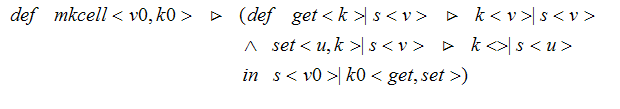# A reference cell in π-calculusExample using reference cell:will receive the value v over the channel d assuming no other processes interacting with the reference cell.

A join-calculus reference cellHere is today's exercise:

1. Modify the π-calculus reference cell so that it behaves like the join-calculus reference cell.

2. Modify the join-calculus reference cell so that it behaves like the π-calculus reference cell.